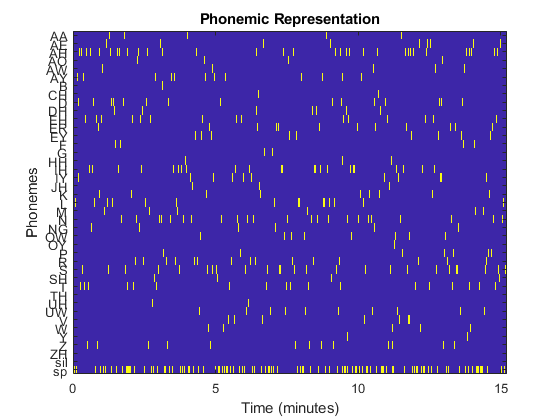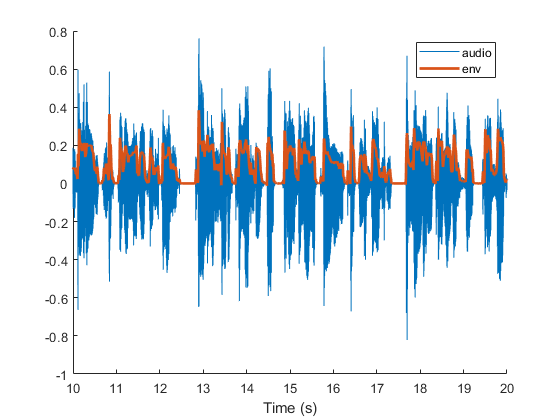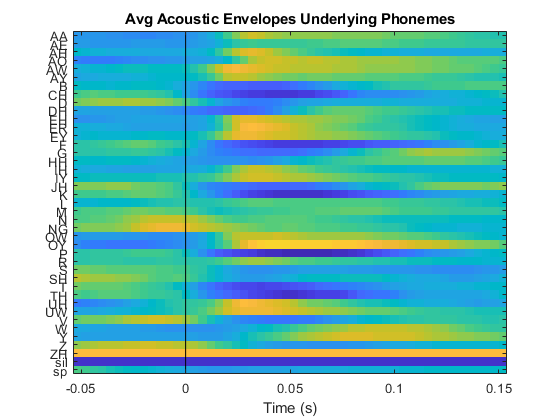# Working with phonemes

This script provides an example for how to prepare phoneme data for use in mTRFs. Here it is assumed the Prosodylab has already been run.

## Go to working directory

```cd C:\Users\WinstonWolfe\CILab\Audiobook\scripts
```

## Importing raw data

```% example struct
ex = [];
% prosodylab time scalar value
ex.plDivisor = 1e7; % use to put pl into seconds
% sampling rate that should match EEG
ex.fs_ph = 250;
% importmlf made by MATLAB import -> generate function + minor changes
ex.data = importmlf('C:\Users\WinstonWolfe\CILab\Audiobook\alignments\new\file4\.aligned.mlf');
ex.data.onset = ex.data.onset/ex.plDivisor; % onset in seconds
ex.data.offset = ex.data.offset/ex.plDivisor; % offset in seconds

% show raw data
ex.table = table(ex.data.onset, ex.data.offset, ex.data.ph, ex.data.word,...
'VariableNames', {'onset', 'offset', 'phoneme', 'word'});
disp(ex.table(1:10,:));

% clean up phonemes
ph = ex.data.ph;
for iph = 1:length(ph)
if ~isnan(str2double(ph{iph}(end)))
% remove last elem and overwrite
ph{iph} = ph{iph}(1:end-1);
end
end
ex.data.ph = ph;

% show cleaned version
ex.data.ph(1:10)
```
```    onset    offset    phoneme      word
_____    ______    _______    _________

0      0.35      "sil"     'sil'
0.35      0.49      "CH"      'CHAPTER'
0.49      0.58      "AE1"     ''
0.58      0.64      "P"       ''
0.64      0.71      "T"       ''
0.71      0.76      "ER0"     ''
0.76      0.76      "sp"      ''
0.76      0.94      "TH"      'THREE'
0.94      1.05      "R"       ''
1.05      1.24      "IY1"     ''

ans =

10×1 string array

"sil"
"CH"
"AE"
"P"
"T"
"ER"
"sp"
"TH"
"R"
"IY"

```

## Getting phoneme data into envelopes

build up the phoneme matrix by finding all instances of a given phoneme and filling up the time slots where each instance occurs with ones.

```nsamps = ceil(ex.data.offset(end)*ex.fs_ph) + 1;
[ex.phUnique, ~, ex.phOrder] = unique(ex.data.ph);

ex.phmat = zeros(length(ex.phUnique), nsamps);

for iph = 1:length(ex.phUnique)
phOccurrences = find(ex.phOrder == iph); % occurrences of ph
timeranges = round(1+ex.fs_ph*[ex.data.onset(phOccurrences) ex.data.offset(phOccurrences)]); % in samples
for occurrence = 1:size(timeranges,1) % for each occurrence of phoneme ph
% write a 1 into the envelope
ex.phmat(iph,timeranges(occurrence,1):timeranges(occurrence,2)) = 1;
end

end

% show phoneme envelopes (phonemes x time)
figure();
imagesc((0:size(ex.phmat,2)-1)/ex.fs_ph/60, 1:size(ex.phmat,1), ex.phmat);
set(gca,'YTick', 1:length(ex.phUnique), 'YTickLabel', ex.phUnique)
title('Phonemic Representation');
xlabel('Time (minutes)');
ylabel('Phonemes');
```## Checking prosodylab alignment consistency

Once you know when each phoneme occurred, the underlying acoustics can be examined by epoching and averaging the audio envelope around different phonemes. The .mlf file read in above was based on audio which we can read in here.

```% read audio
% get envelope
ex.env = abs(hilbert(ex.y));
% filter
[B,A] = butter(4,50/(ex.fs_audio/2), 'low'); % 4th order, 50 Hz cutoff
% downsample to match sampling rate of phonemes
ex.env = resample(filtfilt(B,A,ex.env), ex.fs_ph, ex.fs_audio);
% check envelope
figure();
hold on
plot((0:length(ex.y)-1)/ex.fs_audio, ex.y, 'LineWidth', .5);
plot((0:length(ex.env)-1)/ex.fs_ph, ex.env, 'LineWidth', 2);
legend('audio', 'env');
xlabel('Time (s)');
xlim([10 20]);
```## Extract epochs from the amplitude envelope

```% define epoch window
start = .05; % seconds
fin = .15; % seconds
ex.t = (-round(start*ex.fs_ph):round(fin*ex.fs_ph))/ex.fs_ph; % seconds
% preallocate space for the averaged phomeme acoustics
ex.phacoustics = zeros(size(ex.phmat,1),length(ex.t));
for iph = 1:size(ex.phmat,1) % 41 phonemes
curr_ph = ex.phmat(iph,:); % single phoneme envelope
onsets = find(diff(curr_ph) == 1); % onsets has the sample number when onset occurs
% figure(); hold on; plot(curr_ph); plot(onsets, ones(1,length(onsets)),'*')
epochs = zeros(length(onsets),length(ex.t));
for ionset = 1:length(onsets)
refpoint = onsets(ionset);
try
temp = ex.env(refpoint - round(start*ex.fs_ph): refpoint + round(fin*ex.fs_ph))';
catch
nzeros = abs(refpoint - round(start*ex.fs_ph)) + 1;
temp = [zeros(nzeros,1);ex.env(1: refpoint + round(fin*ex.fs_ph))]';
end
epochs(ionset,:) = temp/max(temp);
end
ex.phacoustics(iph, :) = mean(epochs);
end

% view the average acoustic envelopes
figure()
imagesc(ex.t, 1:size(ex.phmat,1), ex.phacoustics, [0 1]);
set(gca,'YTick', 1:length(ex.phUnique), 'YTickLabel', ex.phUnique)
xlabel('Time (s)');
hold on;
plot([0 0], [0 42], 'k')
title('Avg Acoustic Envelopes Underlying Phonemes');
```